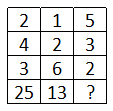Question 1

# In the following question. select the number which can be placed at the sign of question mark (?) from the given alternatives.Solution

In each column, the number at the end is the sum of 1 and product of first three numbers.

Eg : $$(2\times4\times3)+1=24+1=25$$

and $$(1\times2\times6)+1=12+1=13$$

Similarly, $$(5\times3\times2)+1=30+1=31$$

=> Ans - (C)